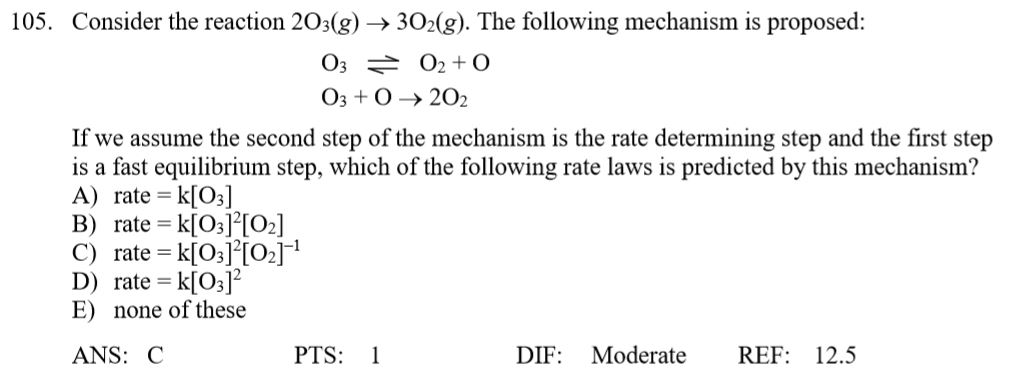# Consider the reaction 2O3(g) → 3 O2(g). The following mechanism is proposed: O3 ⇌ O2 + O O3 + O → 2 O2 If we assume the second step of the mechanism is the rate determining step and the first step is a fast equilibrium step, which of the following rate laws is predicted by this mechanism?